In this post we will describe how we can convert a compressor manufacturer's compressor performance map to a surge line we can use to determine where, in relation to that surge line, a compressor is operating.

Compressor manufacturers present their performance maps as the polytropic head vs. the inlet volumetric flow.

The polytropic head can be expressed with the following equation:Where:

Pd = compressor discharge pressure
Ps = compressor suction pressure
σ = polytropic exponent
= (k-1)/kη
k = specific heat ratio
η = compressor polytropic efficiency
Z = compressibility at suction conditions
R = universal gas constant
T1 = compressor suction temperature
MW = molecular weight of gas

The inlet volumetric flow can be expressed with the following equation: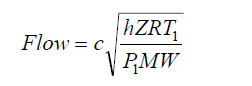Where:

c = flow coefficient of flow meter
h = differential pressure across flow meter

One objective in the development of a surge line prediction algorithm is to simplify these equations if possible. In the forms shown above, no simplification is possible. But if the flow equation is squared, several of the gas terms will cancel out.

The next two figures depict a performance map for a single stage centrifugal compressor.

The surge curve for a single stage compressor is a parabola [Head = f(Flow2)].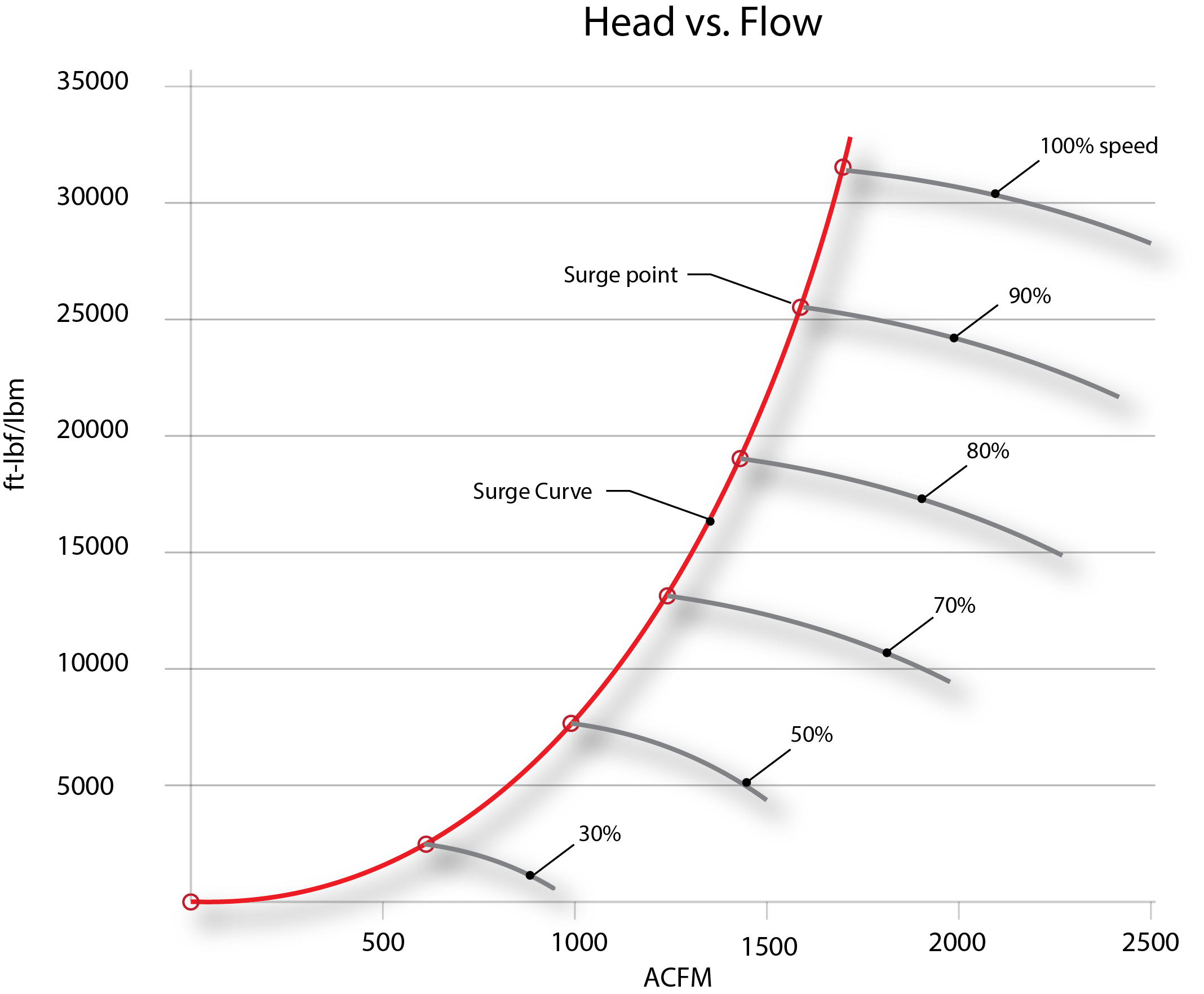Figure 1 - performance map for a single stage centrifugal compressor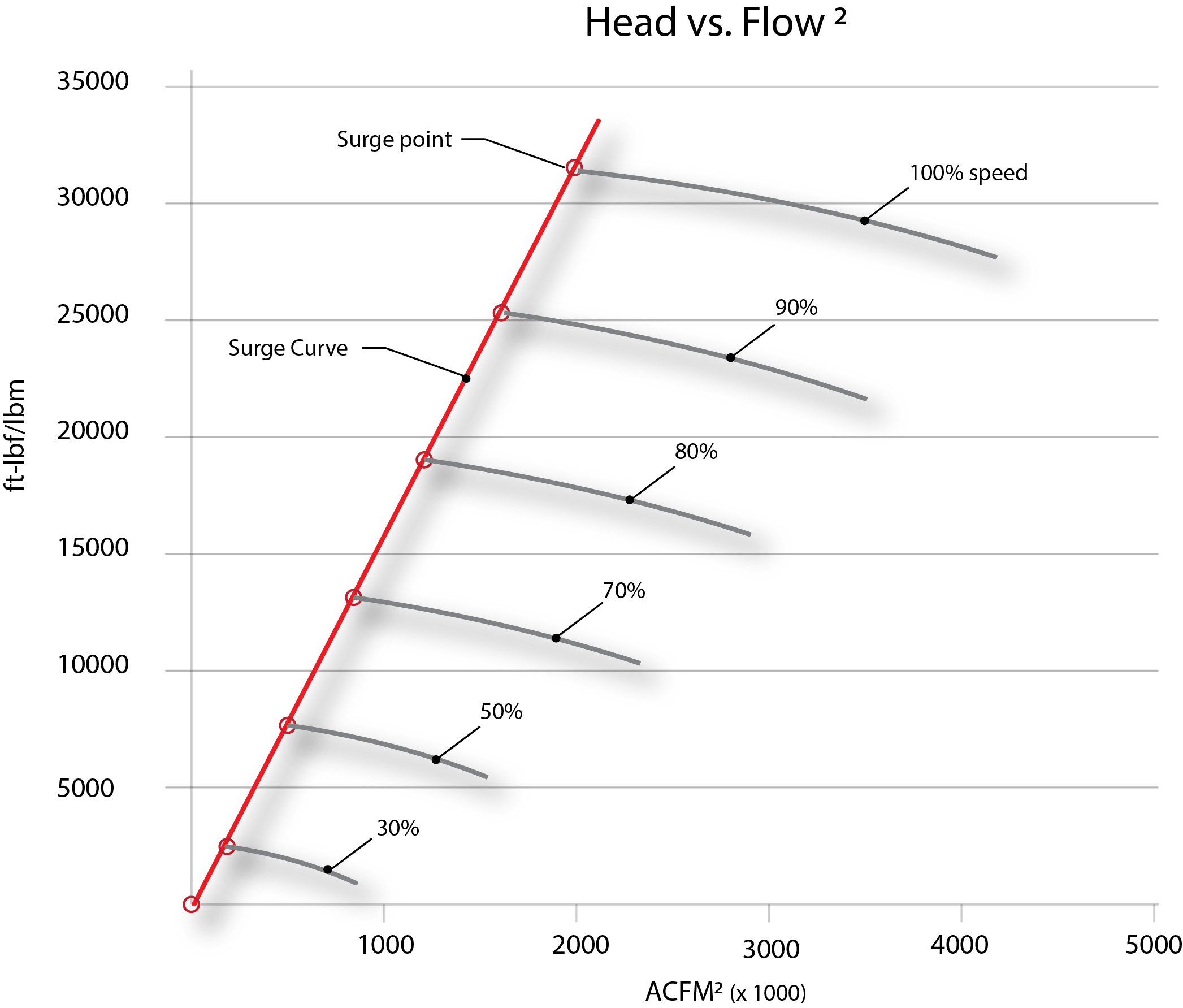Figure 2 -  If the compressor capacity map (from figure 1) is plotted as Head vs. Flow2 the surge curve is linear

When the compressor curve is expressed in terms of the head vs. the flow squared, it is possible to see how the algorithm will reliably predict the surge line even if the gas (molecular weight) changes.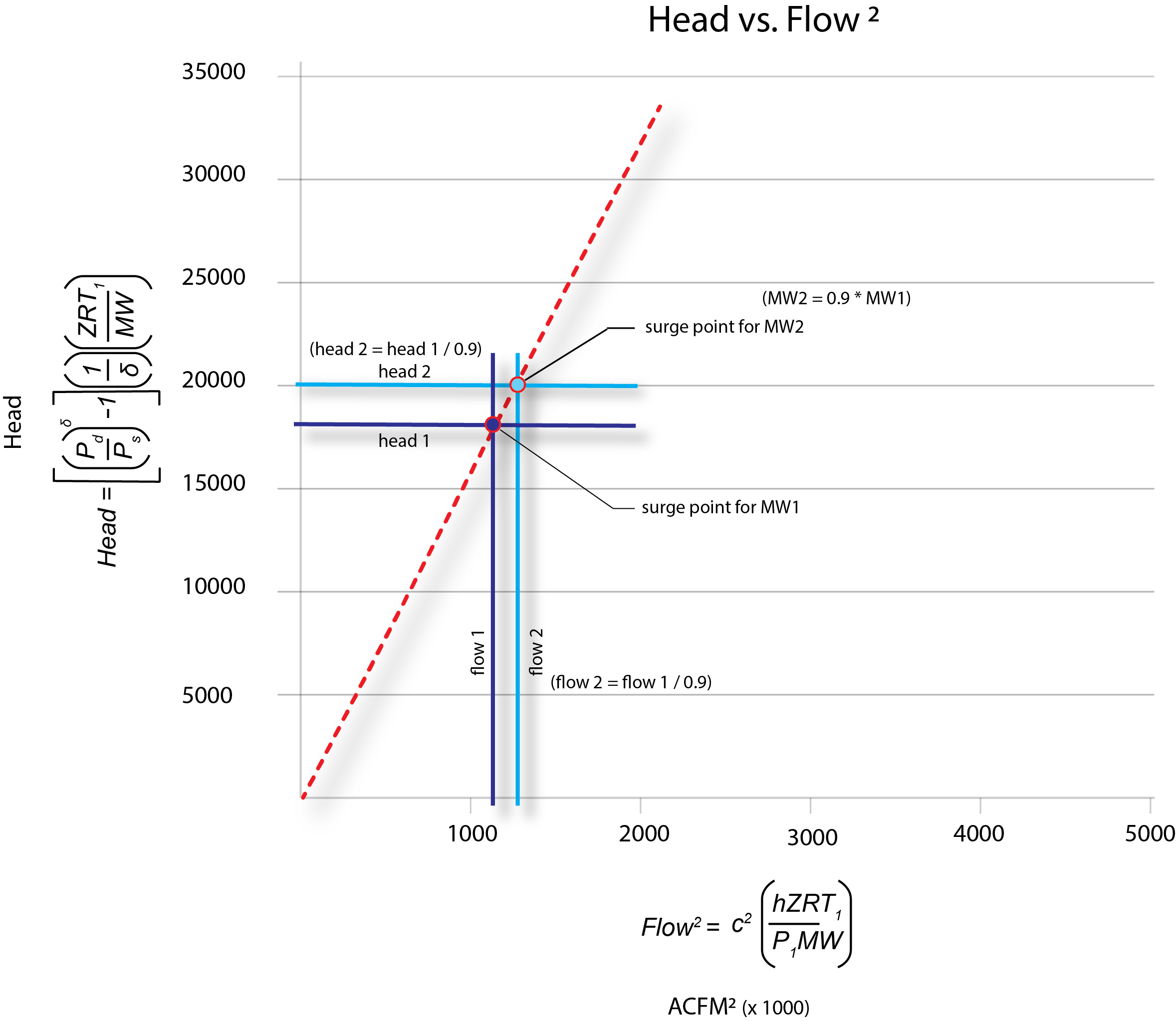Figure 3 – When the surge line is expressed in terms of the head vs. flow squared, any changes
to the molecular weight will affect the head term in the same way that it affects the flow term. So
if the molecular weight drops by 10%, the head will increase by 11% and the flow will also
increase by 11% (1/0.9). The new surge point will fall on the same line.

By cancelling like terms between the head equation and the flow squared equation, the surge
line algorithm reduces to: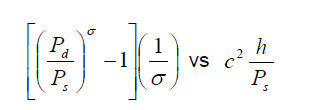The polytropic exponent can be treated as a constant since the specific heat ratio tends to
change very little and the efficiency is constant along the surge line (see figure 5 below). The flow
meter coefficient is a constant, as well. This reduces the surge line algorithm to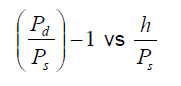If the origin of the map is moved up to a y intercept of 1, the algorithm reduces to: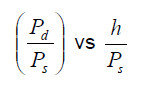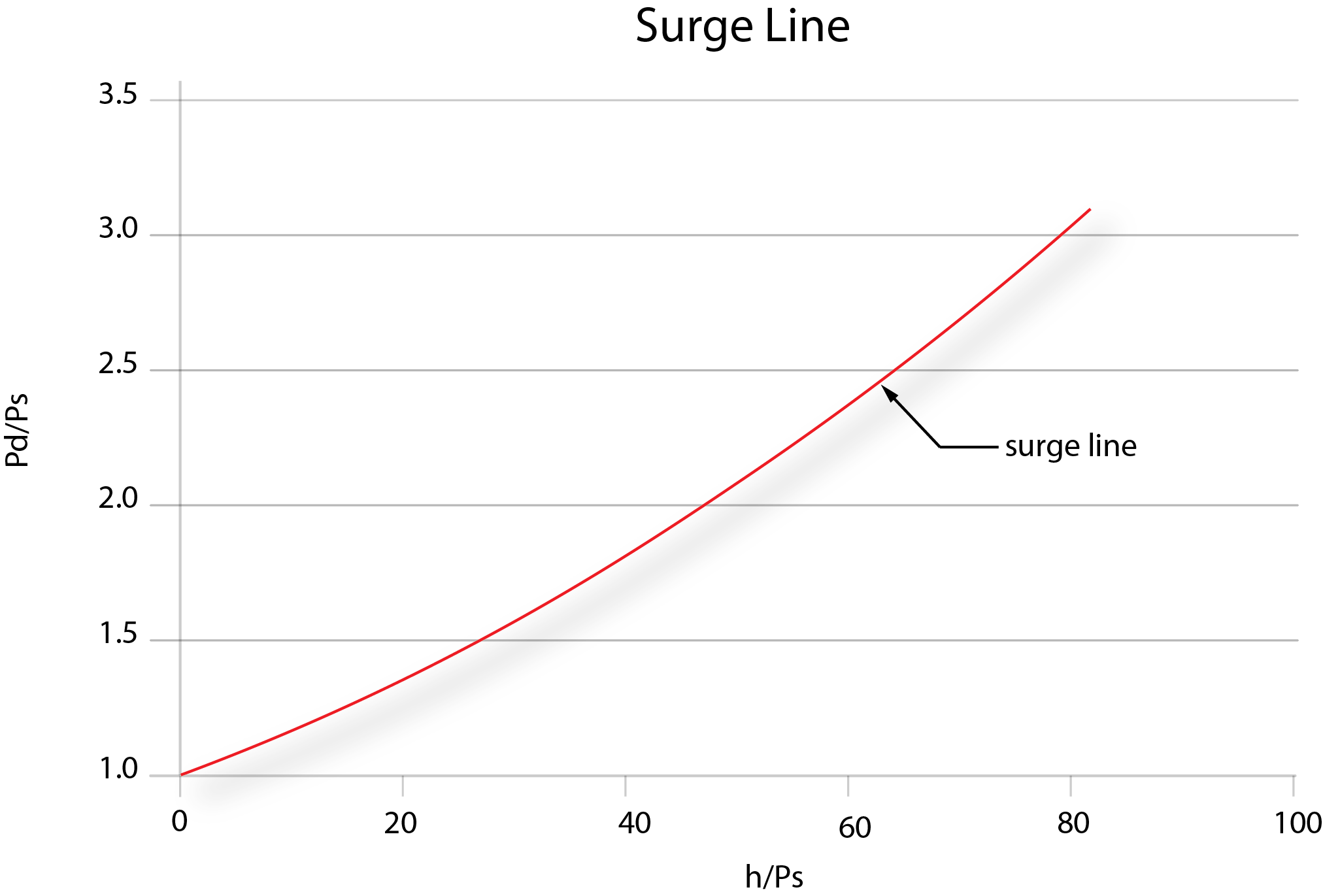Figure 4 – the surge line of the compressor shown in figure 1 becomes the above curve when
our surge curve algorithm is applied.

Efficiency curves – in this example, there is a change in the efficiency at the surge
line over the entire speed range of less than 7%. In the speed range from 70% to 100% (normal
speed range), the deviation is only about ½%. For predicting the surge line, the efficiency can
be assumed constant.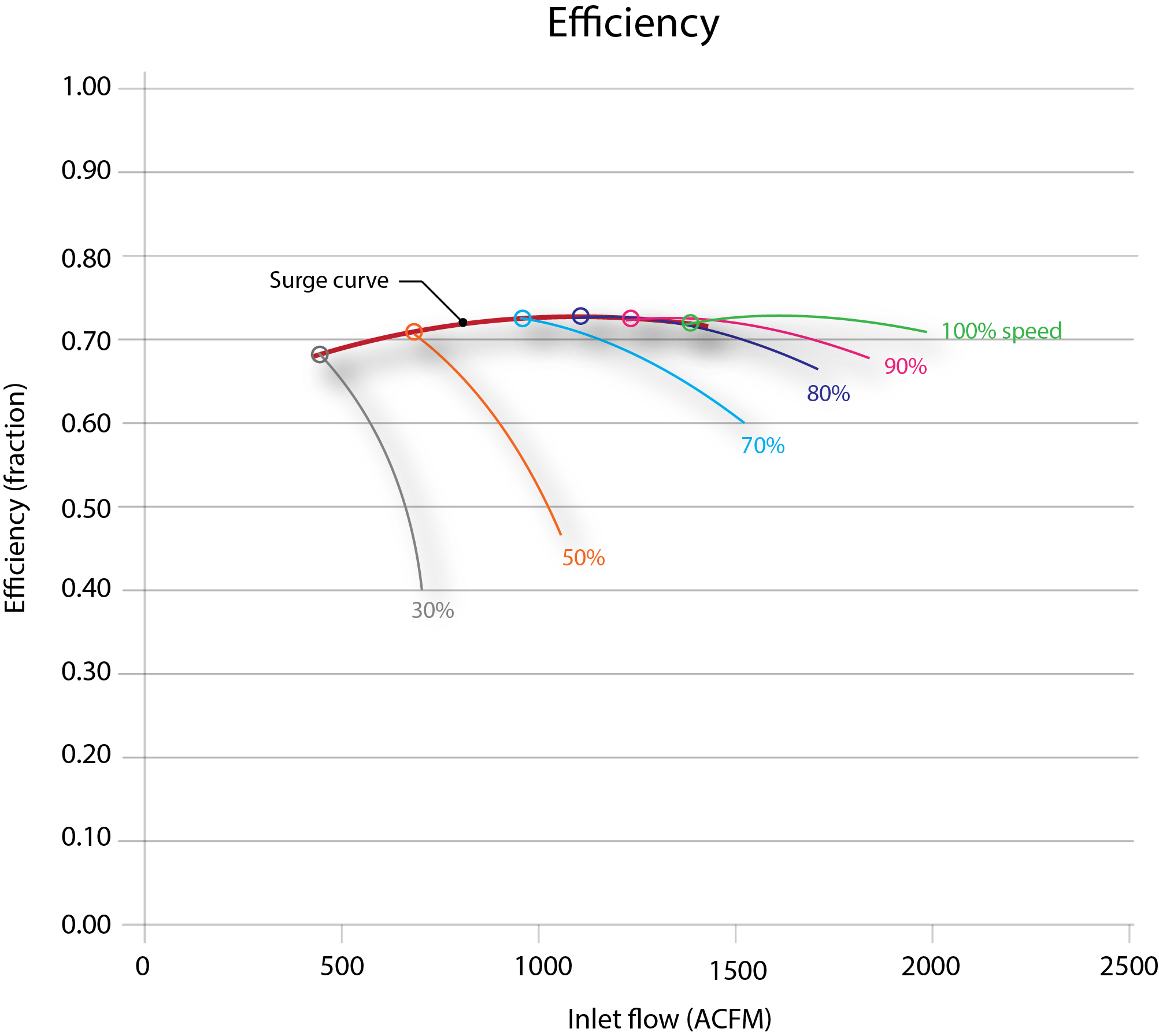# The Tri-Sen Blog...

About once every two weeks, we'll discuss a turbomachinery controls related topic that  we were either asked about recently or a topic that we think you might be interested in learning more about.

Topics that are included or that will be:

• Compressor Controls
• Turbine Controls
• Turbine Safety
• Relevant API directives
• General Industry happenings